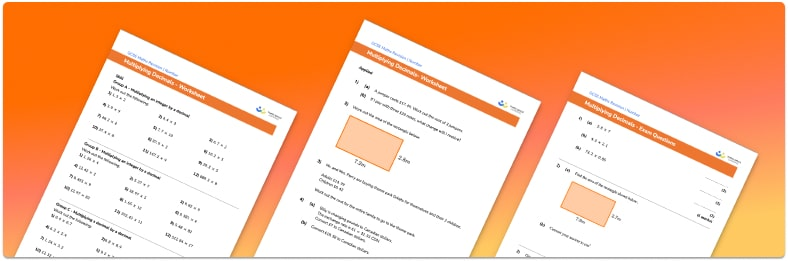# Multiplying Decimals Worksheet• Section 1 of the multiplying decimals worksheet contains 20+ skills-based multiplying decimals questions, in 3 groups to support differentiation
• Section 2 of the decimal multiplication worksheet contains 3 applied multiplying decimals questions with a mix of decimal word problems and deeper problem solving questions
• Section 3 of the multiplying decimal numbers worksheet contains 3 foundation and higher level GCSE exam style multiplying decimals questions
• Answers and a mark scheme for all multiplying decimals questions are provided
• Questions follow variation theory with plenty of opportunities for students to work independently at their own level
• All questions created by fully qualified expert secondary maths teachers
• Suitable for GCSE maths revision for AQA, OCR and Edexcel exam boards

• This field is for validation purposes and should be left unchanged.

You can unsubscribe at any time (each email we send will contain an easy way to unsubscribe). To find out more about how we use your data, see our privacy policy.

### Multiplying decimals at a glance

When doing multiplication of decimals, the first step is to multiply the numbers as if there were no decimal points. For example, for the calculation 3.6 times 1.84, we would begin by calculating multiplying the whole numbers 36 times 184. To do this, we would use an appropriate method for two and three digit multiplication, such as the grid method, giving 36 times 184 = 6624. We then count the total number of decimal places for both decimals. In this case, the total number of decimal places is 1+2=3. We divide the integer answer by 10 that many times. So 6624 div 10^{3} = 6.624.

Knowledge of decimal place values of tenths, hundredths, and thousandths is useful here.

Multiplying decimals is used widely with money and metric conversions.

Looking forward, students can then progress to additional decimals worksheets and other number worksheets, for example a multiplying and dividing decimals worksheet or an order of operations worksheet.For more teaching and learning support on Number our GCSE maths lessons provide step by step support for all GCSE maths concepts.

## Do you have KS4 students who need more focused attention to succeed at GCSE?There will be students in your class who require individual attention to help them succeed in their maths GCSEs. In a class of 30, it’s not always easy to provide.

Help your students feel confident with exam-style questions and the strategies they’ll need to answer them correctly with our dedicated GCSE maths revision programme.

Lessons are selected to provide support where each student needs it most, and specially-trained GCSE maths tutors adapt the pitch and pace of each lesson. This ensures a personalised revision programme that raises grades and boosts confidence.

Find out more